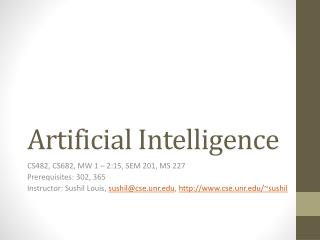# Artificial Intelligence - PowerPoint PPT PresentationDownload PresentationArtificial Intelligence

Artificial IntelligenceDownload Presentation## Artificial Intelligence

- - - - - - - - - - - - - - - - - - - - - - - - - - - E N D - - - - - - - - - - - - - - - - - - - - - - - - - - -
##### Presentation Transcript

1. Artificial Intelligence CS482, CS682, MW 1 – 2:15, SEM 201, MS 227 Prerequisites: 302, 365 Instructor: Sushil Louis, sushil@cse.unr.edu, http://www.cse.unr.edu/~sushil

2. Constraint satisfaction problems • Constraints on the values of variables that define system state • What’s new • State is no longer a black box • Previously all you could do with states was • Test if two states were the same • Tell if a state was a goal state • Now: State space is defined by the values of a set of variables • Each variable’s set of values is the variable’s domain • There can be • Unary • Binary • Path • Constraints

3. CSP • Find values of variables that satisfy all problem constraints • How? • Search, of course • Can we use any search method? • Hmm, some intuition from considering specific problems will help

4. Three colour problem Neighboring regions cannot have the same color Colors = {red, blue, green}

5. Consider using a local search • - 3 to the power 7 possible states = 2187 • But not all states are legal • For example: {r, r, r, r, r, r, r} is NOT legal because it violates our constraint • Suppose we do sequential assignment of values to variables • Assign r (say) to WA then we can immediately reduce the number of possible values for NT and SA to be {g, b}, and if we chose NT = {g}, then SA has to be {b}.

6. Propagation of constraints g r g ?, ?, ? r b b ?, ?, ? ?, ?, ? ?, ?, ?

7. Types • Discrete finite domains • Map coloring, scheduling with time limits • Can enumerate all legal value combinations (that specify constraints) • Discrete infinite domains • Ex: Variable values can be the set of integers • Needs a constraint language to specify constraints • We have solutions for linear constraints over integers • We can prove that no algorithm exists for solving general nonlinear constraints over integers • Continuous domains • Hubble telescope scheduling is continuous over time and must obey a variety of astronomical, precedence, and power constraints • Linear programming  poly time algorithms

8. Types • Unary constraints • Truck height < 14 feet • Binary constraints • WA != SA

9. Cryptarithmetic puzzles

10. Properties • Node consistency (unary) • Arc consistency (binary) • Network arc consistency (all arcs are consistent) • ACS3 is the most popular arc consistency algorithm • Fails quickly if no consistent set of values found • Start: • Considers all pairs of arcs • If making an arc (xi, xj) consistent causes domain reduction • Add all neighboring arcs that go to xi to set of arcs to be considered • Success leaves a much smaller search space for search • Domains will have been reduced • Suppose n variables, max domain size is d, then complexity is O(cd^3) where c is number of binary constraints

11. More constraint types and approaches • Path (triples) • Global constraints (n variables) • Special purpose algorithms (heuristics) • Alldiff constraints (Sudoku) • Remove any variable with singleton domain • Remove that value from the domains of all other variables • Repeat • While • singletons values remain • No domains are empty • Not more variables than domain values • Resource constraints (Ex: Atmost 100) • Bounds and bounds propagation

12. Search • Constraints have been met and propagated • But the problem still remains to be solved (multiple values in domains) • Search through remaining assignments • For CSPs Backtracking search is good • Choose a value for variable, x • Choose a subsequent legal value for next variable, y • Backtrack to x if no legal value found for y

13. Australia coloring

14. Backtracking search algorithm

15. Local search for CSPs

16. SudoKu